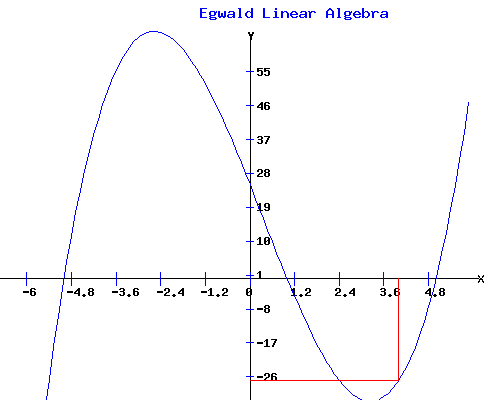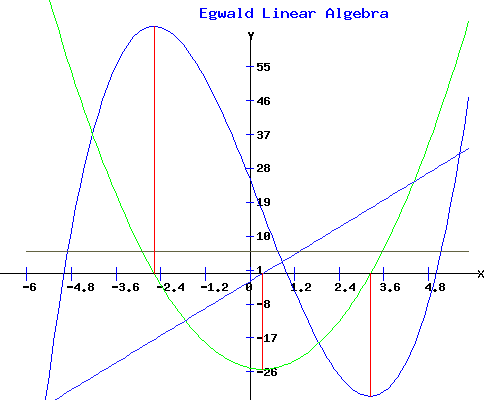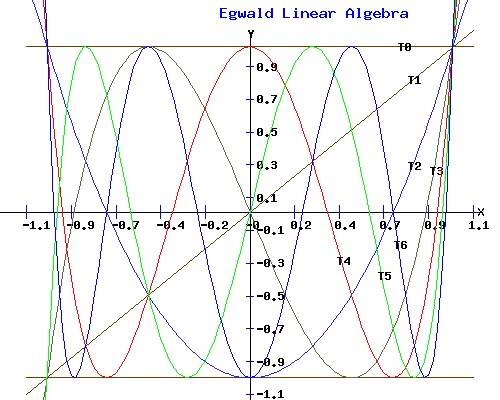Egwald Mathematics: Linear Algebra

Polynomials and Polynomial Roots

by

Elmer G. Wiens

Egwald's popular web pages are provided without cost to users.
Please show your support by joining Egwald Web Services as a Facebook Fan:Follow Elmer Wiens on Twitter:Definition of a Polynomial.

A polynomial is an expression of the form:

p(x) = c1 + c2 * x + c3 * x2 + .... + cn+1 * xn

where the coefficients c1, c2, ..., cn+1 are given real or complex numbers, and the variable x takes on takes on real or complex number values. A single term of the form ck * xk+1 is called a monomial.

The degree of the polynomial p(x) is n if cn+1 is not zero. The nonzero coefficient with the highest power of x is the leading coefficient.

For example, the polynomial:

p(x) = 25 - 25 * x - 1 * x2 + 1 * x3

is of degree 3, with 4 real number coefficients. If I evaluate the polynomial p(x) at x = 4:

y = p(4) = 25 - 25 * 4 - 1 * 42 + 1 * 43 = -27

The graph of the polynomial p(x), between -6 and 6, is displyed below.The values of x at which p(x) takes on the value of zero, i.e. where the graph of p(x) crosses the x-axis, are called the roots of the polynomial p(x). The roots of p(x) are -5, 1, and 5.

Constant Polynomial (Functions).

A constant polynomial is of degree 0 since all terms but c1 are zero. For example, the polynomial:

c(x) = c1 = 6

is a constant polynomial. By convention, the zero polynomial with c1 = 0 has no degree.

Linear Polynomial (Function).

Linear polynomials have the form l(x) = c1 + c2 * x, and are of degree 1. For example, the polynomial:

l(x) = c1 + c2* x = - 2 + 6 * x

is a linear polynomial.

The fundamental theorem of algebra states that any polynomial p(x) of degree n can be factored into the product of n linear polynomials, some of whose coefficients may be complex numbers.

Quadratic Polynomial (Function).

Quadratic polynomials have the form q(x) = c1 + c2 * x + c3 * x2. For example, the polynomial:

q(x) = c1 + c2* x+ c2 * x + c3 * x2 = - 25 - 2 * x + 3 * x2

is a quadratic polynomial.

Any polynomial of degree n with real coefficients can be factored into the product of quadratic polynomials with real coefficients, times one linear polynomial with real coefficients if n is an odd number.

The graph of an arbitrary quadratic can be obtained by setting the coefficients in the form that follows, and clicking "Graph".

Graph of the quadratic p(x)
p(x) = x0 + x1 + x2Cubic Polynomial (Function).

Cubic polynomials have the form p(x) = c1 + c2 * x + c3 * x2 + c4 * x3. For example, the polynomial:

p(x) = c1 + c2* x+ c2 * x + c3 * x2 + c4 * x3 = -25 - 2 * x + 3 * x2 + 5 * x3

is a cubic polynomial.

The graph of an arbitrary cubic can be obtained by setting the coefficients in the form that follows, and clicking "Graph".

Graph of the cubic p(x)
p(x) = x0 + x1 + x2 + x3Biquadratic Polynomial (Function).

Biquadratic polynomials have the form p(x) = c1 + c2 * x + c3 * x2 + c4 * x3 + c5 * x4. For example, the polynomial:

p(x) = c1 + c2* x+ c2 * x + c3 * x2 + c4 * x3 + c5 * x4 = -20.25 + 27 * x + -4.5 * x2 + -3 * x3 + 1 * x4

is a biquadratic polynomial.

The graph of an arbitrary biquadratic can be obtained by setting the coefficients in the form that follows, and clicking "Graph".

Graph of the biquadratic p(x)
p(x) = x0 + x1 + x2 + x3 x4Derivatives of Polynomials.

Polynomials are easy to differentiate. The derivative of the polynomial p(x) is another polynomial q(x) given by:

q(x) = p'(x) = c2 + 2 * c3 * x + 3 * c4 * x2 + ... + N * cN+1 * xN-1.

The derivative of a polynomial is a polynomial of degree 1 less than the degree of the original polynomial.

PolynomialsRootsFactors
p(x) = 25 - 25 * x - 1 * x2 + 1 * x3 x1 = -5, x2 = 1, x3 = 5-1*(-5 - x), (1 - x), (5 - x)
q(x) = p'(x) = -25 - 2 * 1 * x + 3 * 1 * x2x1 = -2.5726, x2 = 3.23933*(-2.5726 - x), (3.2393 - x)
l(x) = q'(x) = -2 + 2 * 3 * x = -2 + 6 * x x1 = 1/3-6*(1/3 - x)
c(x) = l'(x) = 6
c'(x) = 0If one examines the graphs of the polynomials in the preceeding diagram, one sees that the graph of the derivative of a polynomial descends through the x-axis at that value of x where the original polynomial has a (local) maximum; it ascends through the x-axis at that value of x where the original polynomial has a (local) minimum.

Rolle's Theorem.

Between two consecutive real roots x1 and x2 of a polynomial p(x), its derivative polynomial p'(x) has at least one, or a greater odd number of roots. Furthermore, between two consecutive real roots x3 and x4 of p'(x), p(x) has at most one root.

Adding Polynomials.

Given two polynomials, p(x) of degree n, and f(x) of degree m, where without loss of generality (W.L.O.G.) m < n:

p(x) = c1 + c2 * x + c3 * x2 + .... + cn+1 * xn

f(x) = d1 + d2 * x + d3 * x2 + .... + dm+1 * xm

their sum is given by:

p(x) + f(x) = (c1 + d1) + (c2 + d2) * x + (c3 + d3) * x2 + ... + (cm+1 + dm+1) * xm + . . . + cn+1 * xn

For example, if

p(x) = 25 - 25 * x - 1 * x2 + 1 * x3

f(x) = -25 - 2 * x + 3 * x2

their sum is given by:

p(x) + f(x) = (25 - 25) + (-25 - 2) * x + (-1 +3) * x2 + 1 * x3 = -27 * x + 2 * x2 + 1 * x3

Multiplying Polynomials.

The product of two polynomials p(x) and f(x)is given by:

p(x) * f(x) = (c1 * d1) + (c1 * d2 + c2* d1) * x + (c3 * d1 + c2 * d2 + c1 * d3) * x2 + ... + (cn-m+1 * dm+1 + ... + cn * d2 + (cn+1 * d1) * xn + ... + cn+1 * dm+1 * xn+m

For example, with p(x) = 25 - 25 * x - 1 * x2 + 1 * x3, and f(x) = -25 - 2 * x + 3 * x2, then p(x) * f(x) is found by:

 25 - 25 * x - 1 * x2 + 1 * x3 -25 - 2 * x + 3 * x2 --------------------------------- -625 + 625 * x + 25 * x2 - 25 * x3 -50 * x + 50 * x2 + 2 * x3 - 2 * x4 75 * x2 - 75 * x3 - 3 * x4 + 3 * x5 ---------------------------------------------------------------------- -625 + 575 * x + 150 * x2 - 98 * x3 - 5 * x4 + 3 * x5

Dividing Polynomials.

If polynomials p(x), the dividend, and f(x), the divisor, are related by the formula:

p(x) = f(x)*q(x) + r(x)

where the q(x) is of degree (n-m), then q(x) is the quotient polynomial, and r(x) is the remainder polynomial.

For example, with p(x) = 25 - 25 * x - 1 * x2 + 1 * x3, and f(x) = -25 - 2 * x + 3 * x2, then p(x) / f(x) is:

p(x) = f(x) * (-1/9 + 1/3 * x) + (200/9 - 152/9 * x)

where q(x) = -1/9 + 1/3 * x, and r(x) = 200/9 - 152/9 * x.

Dividing polynomials by hand is a pain. With the form below, you can compute p(x) / f(x) for p(x) and f(x) up to degree 6. Just set their coefficients, and click "Divide". Be sure that the degree of p(x) >= degree of f(x). Leading zero coefficients are ignored.

Division of polynomials: p(x) / f(x)
p(x) = x0 + x1 + x2 + x3 + x4 + x5 + x6
f(x) = x0 + x1 + x2 + x3 + x4 + x5 + x6

p(x) = f(x)*q(x) + r(x)
q(x) = 16 * x0 + 11 * x1 + 4 * x2 + 1 * x3
r(x) = -17 * x0 + -75 * x1

The Remainder Theorem.

The remainder after p(x) is divided by (a - x) is p(a):

p(x) = (a - x)*q(x) + r(x), implies

p(a) = (a - a)*q(x) + r(a) = r(a)

Polynomial Roots.

If x1 is a root of the polynomial p(x) of degree n, ie p(x1) = 0, then by the remainder theorem, p(x) is divisible by (x1 - x):

p(x) = (x1 - x) p1(x)

with p1 of degree n-1.

If x2 is another root of p(x) different from x1, then

p(x2) = (x1 - x2) p1(x2) = 0

implies that

p1(x2) = 0

Hence, p1(x) is divisible by (x2 - x). Therefore,

p1(x) = (x2 - x) p2(x)

for some p2(x) of degree n-2. Consequently,

p(x) = (x1 - x)(x2 - x) p2(x) = [x1*x2 - (x1 + x2) * x + x2] * p2(x).

This procedure can be repeated, factoring the polynomial p(x) into the product of linear and/or quadratic polynomials.

The last equation provides some interesting information. The product of the roots of a quadratic equation equals the c1 coefficient; and, their sum equals the negative of the c2 coefficient. Furthermore, if a quadratic with real coefficients has a complex root, x1 = a + b*i, then its other root must be x2 = a - b*i, the complex conjugate of x1. Then, x1*x2 = a2 + b2; and, x1 + x2 = 2*a.

Examples with p(x) as a cubic polynomial.

p(x) = -30 + 11 * x + 4 * x2 - 1 * x3 = (-3 - x)(2 - x)(5 - x) = [-6 + 1 * x + 1 * x2] * (5 - x)

p(x) = 25 + 15 * x + 1 * x2 - 1 * x3 = (-2+1*i - x)(-2-1*i - x)(5 - x) = [5 + 4 * x + 1 * x2] * (5 - x)

Finding a Polynomial's Roots.

Since any polynomial p(x) of degree n >= 2 with real coefficients can be written as:

p(x) = [c1 + c2 * x + 1* x2] * p1(x) + r(x),

search for real numbers c1 and c2 so that r(x) = 0. Then two roots of p(x) are:

x1 = (-c2 + sqrt(c22 - 4*c1)) / 2,

x2 = (-c2 - sqrt(c22 - 4*c1)) / 2.

After dividing p(x) by the quadratic q(x) = c1 + c2 * x + 1* x2, repeat this process with p1(x), etc., until all the roots of p(x) are obtained.

I use the procedure described above to find the roots of an arbitrary polynomial of up to degree 6. Enter the polynomial's coefficients in the form below and click "Roots". The new polynomial's roots and graph will display.

Find the roots of the polynomial p(x)
p(x) = x0 + x1 + x2 + x3 + x4 + x5 + x6

Roots of p(x)
root 1root 2root 3root 4root 5

-0

4

2 - 3*i

2 + 3*i

-3Alternate Representations of Polynomials.

Polynomials can be expressed in the following forms

 p(x) = c1 + c2 * x + c3 * x2 + .... + cn+1 * xn p(x) = c0 + c1 * x + c2 * x2 + .... + cn * xn p(x) = cn+1 * xn + cn * xn-1 + .... + c2 * x + c1 p(x) = cn * xn + cn-1 * xn-1 + .... + c1 * x + c0 p(x) = c0 * xn + c1 * xn-1 + .... + cn * x + cn+1

Chebyshev Polynomials.

Chebyshev Polynomials can be used to approximate an arbitrary function f(x). Easy to perform operations, such as integration or differentiation, on the Chebyshev Polynomials replace operations on the function f(x).

The first six Chebyshev Polynomials, and their general forms are:

 T0(x) = 1 T1(x) = x T2(x) = 2*x2 - 1 T3(x) = 4*x3 - 3*x T4(x) = 8*x4 - 8*x2 + 1 T5(x) = 16*x5 - 20*x3 + 5*x T6(x) = 32*x6 - 48*x4 + 18*x2 - 1         . . . . . . . . . . Tn+1(x) = 2*x*Tn(x) - Tn-1(x) Tn(x) = cos(n * arcos(x))

Notice that in the following diagram all roots of each Tn(x) polynomial occur in the [-1,1] interval of the x-axis. This holds true for each positive interger n. Furthermore, the range of each Tn(x) is [-1, 1] over the [-1, 1] domain.References.

• Ayres, Frank Jr. Modern Algebra. New York: Schaum McGraw-Hill, 1965.
• Burden, Richard L. and J. Douglas Faires. Numerical Analyis. 6th ed. Pacific Grove: Brooks/Cole, 1997.
• Miller, Norman, and Robert E. K. Rourke. An Advanced Course in Algebra. Toronto: MacMillan, 1941.
• Press, William H., Brian P. Flannery, Saul A. Teukolsky, and William T. Vetterling. Numerical Recipes: The Art of Scientific Computing. Cambridge: Cambridge UP, 1989.
• Uspensky, J. V. Theory of Equations. New York: McGraw-Hill, 1948.Copyright © Elmer G. Wiens:   EgwaldWeb ServicesAll Rights Reserved.    Inquiries